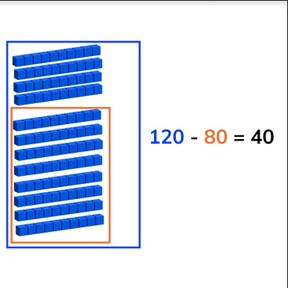Subtraction through 100 using tens

# Subtraction through 100 using tens

No account needed.8,000 schools use Gynzy92,000 teachers use Gynzy1,600,000 students use Gynzy

## General

Students learn to subtract numbers through 100 with tens.

2.NBT.B.5
2.OA.A.1

## Relevance

It is important to be able to subtract beyond 100 with tens, so you know how many you have left.

## Introduction

Name a number that is close to a hundreds number. Tell students that they will count forwards as a class but that they can't discuss who will say which number. If two students say the same number, restart and give the class a new number. Repeat counting backwards.

## Development

Start by refreshing with students that tens are a group of ten and always end with a 0 in the ones place. The learning goal is shown using visual support, using abstract numbers, and as story problems. You can navigate between these methods using the blue menu on the bottom right to select the method best suited to your classroom. Next explain that you can subtract by counting amounts. You start by counting how many objects there are in total, this is the first number of your subtraction problem (the minuend). Next you count how many objects are taken away, this becomes the second number of your subtraction problem (the subtrahend). When you take the second number from the first you are left with the difference. Show this on the interactive whiteboard using the blocks. Check that students are able to do this by asking them to subtract the blocks. Next tell students that you can subtract different problems in different ways. Explain that they can use a number line. They can also choose to split the second number(the subtrahend) into two and subtract back to 100 first, and then to take away the rest. You can also decide to take away the second number in one step. Emphasize that you must carefully look at the numbers in the subtraction problem to determine the best way to subtract the given numbers. Ask students to solve a few subtraction problems and discuss their strategy. Make sure that students are aware that all strategy is valid- it has to do with what makes the subtraction problem easier for them to solve. Finally discuss with students how to solve story problems. Have students determine what kind of math problem is being told, which numbers are required for the math problem, to say or write the problem, and finally to solve the math problem. Do one story problem as a class, and then ask students to solve some story problems individually or in pairs.

Check that students are able to subtract numbers through 100 by asking the following questions:
- What steps do you take to solve a subtraction problem on a number line?
- What steps do you take to solve a story problem?
- How do you solve 130 - 80 and 160 - 90?

## Guided practice

Students start by subtracting numbers with visual support, they then are given problems in the abstract, and finally are asked to solve a story problems.

## Closing

Discuss with students that it is important to be able to subtract through 100 using tens so that you can determine how much you have left. On the interactive whiteboard, show the blocks and the accompanying subtraction problem. Ask students to determine the difference. Repeat this for the abstract problem and the story problem. Ask students to write their answers on a sheet of paper/mini whiteboard to check their work by erasing the grey square on the interactive whiteboard.

## Teaching tips

Students who have difficulty with this learning goal can be supported by the use of a number line in solving their subtraction problems. Remind students that they can split the subtrahend into (many) smaller numbers and jump those smaller steps on the number line.

### The online teaching platform for interactive whiteboards and displays in schools

• Save time building lessons

• Manage the classroom more efficiently

• Increase student engagement